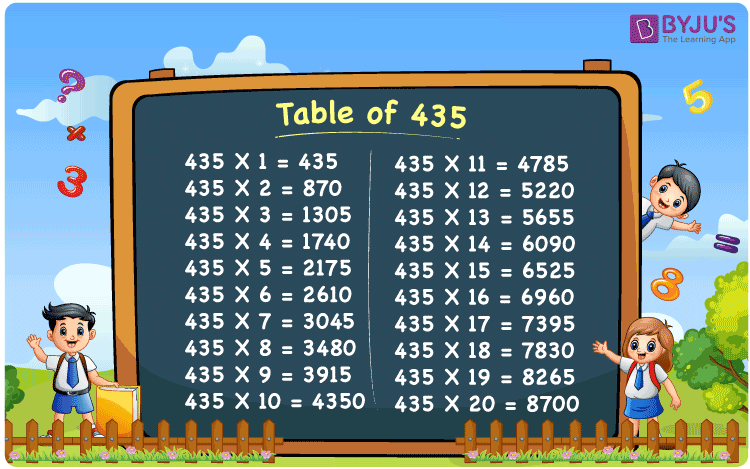Checkout JEE MAINS 2022 Question Paper Analysis : Checkout JEE MAINS 2022 Question Paper Analysis :

# Table of 435

The table of 435 is the multiplication of 435 by natural numbers and it can be written as 435 × 1 = 435, 435 × 2 = 870, 435 × 3 = 1305, 435 × 4 = 1740, 435 × 5 = 2175, etc. We know that multiplication tables can also be created through repeated addition. In this article, you will learn the table of 435 with the help of a chart and example. Also, download the PDF of the 435 times table using the link given below:

## Table of 435 Chart## What is the 435 Times Table?

The 435 times table is also called the addition table for 435 since it can be formed from the repeated addition of 435 as many times as required. Below is the 435 times tables up to 10 times.

 Using multiplication Using repeated addition 435 × 1 = 435 435 435 × 2 = 870 435 + 435 = 870 435 × 3 = 1305 435 + 435 + 435 = 1305 435 × 4 = 1740 435 + 435 + 435 + 435 = 1740 435 × 5 = 2175 435 + 435 + 435 + 435 + 435 = 2175 435 × 6 = 2610 435 + 435 + 435 + 435 + 435 + 435 = 2610 435 × 7 = 3045 435 + 435 + 435 + 435 + 435 + 435 + 435 = 3045 435 × 8 = 3480 435 + 435 + 435 + 435 + 435 + 435 + 435 + 435 = 3480 435 × 9 = 3915 435 + 435 + 435 + 435 + 435 + 435 + 435 + 435 + 435 = 3915 435 × 10 = 4350 435 + 435 + 435 + 435 + 435 + 435 + 435 + 435 + 435 + 435 = 4350

## Multiplication Table of 435

The multiplication table of 435 is given below to help you learn the table of 435 up to 20 times.

 435 × 1 = 435 435 × 2 = 870 435 × 3 = 1305 435 × 4 = 1740 435 × 5 = 2175 435 × 6 = 2610 435 × 7 = 3045 435 × 8 = 3480 435 × 9 = 3915 435 × 10 = 4350 435 × 11 = 4785 435 × 12 = 5220 435 × 13 = 5655 435 × 14 = 6090 435 × 15 = 6525 435 × 16 = 6960 435 × 17 = 7395 435 × 18 = 7830 435 × 19 = 8265 435 × 20 = 8700

## Solved Example

Question:

The price of a hat is Rs. 435. What will be the amount to be paid for 12 such hats?

Solution:

Given,

The price of 1 hat = Rs. 435

Number of hats = 12

Total amount to be paid = ?

Using the table of 435, 435 × 12 = 5220.

Thus, the total amount to be paid for 12 hats = Rs. 435 × 12 = Rs. 5220.

## Frequently Asked Questions on Table of 435

### What is the table of 435?

The table of 435 is also called the 435 times table or the multiplication table of 435. In maths, the multiplication table for 435 is 435 × 1 = 435, 435 × 2 = 870, 435 × 3 = 1305, 435 × 4 = 1740, and so on.

### What is the result of 435 times 7?

Using the table of 435, the value of 435 times 7 = 435 × 7 = 3045.

### How many times of 435 is 6525?

Fifteen times of 435 or 435 times 15 is 6525, i.e. 435 × 15 = 6525.